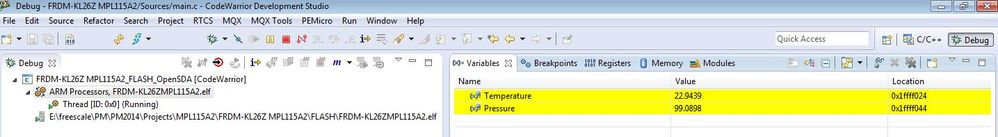## pressure sensor MPL115A2 with FRDM-KL26Z

cancel
Showing results for
Did you mean:
SOLVED

## pressure sensor MPL115A2 with FRDM-KL26Z

453 ViewsContributor II

HI, I am working on a demo on smart inhaler. Does anyone have the source code for pressure sensor MPL115A2? I am trying to use it with FRDM-KL26Z. Any help on where can I find it would be appreciated.

Labels (1)
• ### Pressure Sensors

Tags (3)
1 Solution
182 ViewsNXP TechSupport

Hi Parth,

Attached you can find the source code for the FRDM-KL26Z board and the MPL115A2 pressure sensor on the KITMPL115A2I2C board. It is written in the CW for MCU's v10.6 and uses the I2C1 module (PTC1 and PTC2 pins) for I2C communication. Both the pressure and temperature are calculated in the infinite while loop:

```while(1)
{
I2C_WriteRegister(MPL115A2_I2C_ADDRESS, CONVERT_REG, 0x00);        // Start pressure and temperature conversion

Pause(0x17B2);        // Wait ~4ms (3ms is a minimum delay)

// Temperature calculation
Tadc = ((uint16_t) (RawPressureTempData<<8 | RawPressureTempData)) >> 6;
Temperature = (605.75 - Tadc) * 0.186916;

// Pressure calculation
Padc = ((uint16_t) (RawPressureTempData<<8 | RawPressureTempData)) >> 6;
c12x2 = (((float) C12) / 4194304) * Tadc;
a1 = (((float) B1) / 8192) + c12x2;
y1 = (((float) A0) / 8) + a1x1;
a2x2 = (((float) B2) / 16384) * Tadc;
Pcomp = y1 + a2x2;
Pressure = (Pcomp * 65 / 1023) + 50;

Pause(0xFFFF);
}

```

and can be watched in the "Variables" window on the top right of the Debug perspective:I hope it helps.

Regards,

Tomas

2 Replies
183 ViewsNXP TechSupport

Hi Parth,

Attached you can find the source code for the FRDM-KL26Z board and the MPL115A2 pressure sensor on the KITMPL115A2I2C board. It is written in the CW for MCU's v10.6 and uses the I2C1 module (PTC1 and PTC2 pins) for I2C communication. Both the pressure and temperature are calculated in the infinite while loop:

```while(1)
{
I2C_WriteRegister(MPL115A2_I2C_ADDRESS, CONVERT_REG, 0x00);        // Start pressure and temperature conversion

Pause(0x17B2);        // Wait ~4ms (3ms is a minimum delay)

// Temperature calculation
Tadc = ((uint16_t) (RawPressureTempData<<8 | RawPressureTempData)) >> 6;
Temperature = (605.75 - Tadc) * 0.186916;

// Pressure calculation
Padc = ((uint16_t) (RawPressureTempData<<8 | RawPressureTempData)) >> 6;
c12x2 = (((float) C12) / 4194304) * Tadc;
a1 = (((float) B1) / 8192) + c12x2;
y1 = (((float) A0) / 8) + a1x1;
a2x2 = (((float) B2) / 16384) * Tadc;
Pcomp = y1 + a2x2;
Pressure = (Pcomp * 65 / 1023) + 50;

Pause(0xFFFF);
}

```

and can be watched in the "Variables" window on the top right of the Debug perspective:I hope it helps.

Regards,

Tomas